# Why #NULL! occur And How to Fix #NULL! in Excel

In this article, we will learn about why #NULL! occur And How to Fix #NULL! in Excel.

In excel, at times we get #NULL! error. This error occurs when there is something important missing with range which can't be identified in the formula you have supplied to the cell and is recognized by Excel by showing the error. As per Microsoft official site a #NULL! is Excel's way of saying, the range supplied is not valid in the formula you provided.

If you are using cell reference to array, if it's not in valid format this error suggests you to check some points discussed below. This usually happens when you try to manually type the formula and wrong character is used instead of the required character.

Let’s understand how this error occur and how to fix them with some examples. First of all check the formula in the cell for each and every character carefully.

## #NULL! error range not provided in valid format.

Wrong character instead of colon ( : )

The most basic reason of #NULL! error is when the formula used in the cell doesn't support the excel format. Generally Excel has predefined format for the formula which it accepts only if that format is followed. Here #NULL! error suggests that array provided as cell reference is not followed by the correct format. See the below example to understand more.

For example, the SUM function adds up all the values given in as input. Here the range (A2:A21) to add up is provided as cell reference.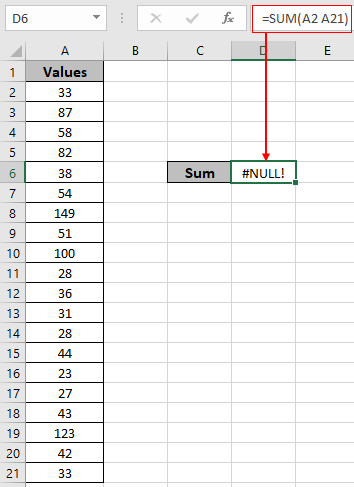Look in the above snapshot and realize the mistake just happened. The above formula has space character instead of Colon ( : ) character between two cell reference ( A2 & A21 ).

How to fix it!

You can fix the error for the result. See the below format type you can use in excel. See the following excel correct format shown below.

First Method:

Select and drop down till required cell. Using cell reference correct format for providing range in the formula.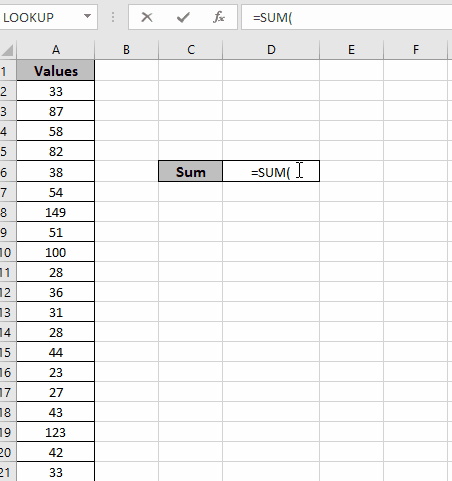In the above gif, the range is provided. Follow the below explained steps to avoid errors.

1. First type the function name and open bracket symbol. =SUM(
2. Then left click on mouse where the cells range starts and do not leave the mouse left click.
3. Till the required cell and press Enter to view the result. You will see the colon character used in the range is default with this process.

Second Method:

Using the named range option in excel. Name the range using the named range attribute of excel. In the gif below shown, is a type of named range.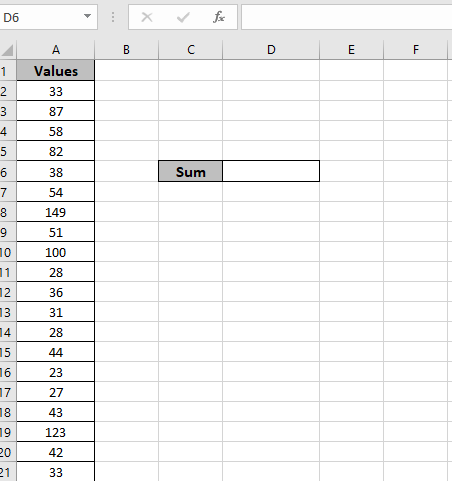In the above gif named range has been assigned and used in the formula. Follow the below steps to avoid having #NULL error.

1. First, select the range and name the range values via typing the values in the top left box.
2. Secondly, type the function name and a open bracket symbol. =SUM(
3. Then type the exact name of the named range (  ) as it is case sensitive.
4. Excel will show you the suggestion on the named range as you start typing the correct name for the range.
5. Press Enter to view the SUM.

As you can see the #NULL! Error can be handled when you use correct Excel format while using range in the formula.

## #NULL! error cell references not provided in valid format.

Wrong character instead of comma ( , )

The most basic reason of #NULL! error is when the formula used in the cell doesn't support the excel format. Generally, Excel has a predefined format for the formula which it accepts only if that format is followed. Here #NULL! error suggests that cells provided as cell references is not followed by the correct format. See the below example to understand more.
For example, the SUM function adds up all the values given in as input. Here all the individual cell values needed to be added.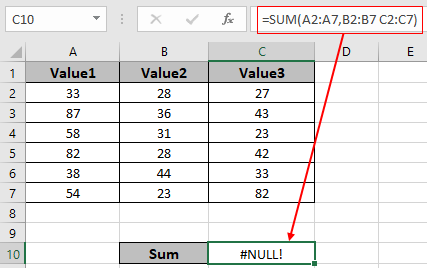Look in the above snapshot and realize the mistake just happened. The above formula has space character instead of Colon ( , ) character between two cell references ( B2:B7 & C2:C7 ).

How to fix it!

You can fix the error for the result. See the below type format you can use in excel. See the following excel correct format shown below.

First Method:

Use Comma character ( , ) every time when two cell references or named references are used in the same formula. Comma character ( , ) is understood by excel as two different ranges references.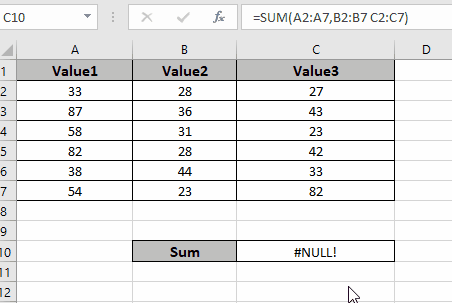In the gif shown above, the range is provided with the correct format to the function. Follow the steps explained below to avoid errors.

1. First double click the formula cell and edit the formula.
2. Then remove the extra space character.
3. Use the Comma Character ( , ) between two cell references ( B2:B7 & C2:C7 )

Second Method:

Using the named range option in excel. Name the range using the named range attribute of excel. In the below gif shown is a type of named range.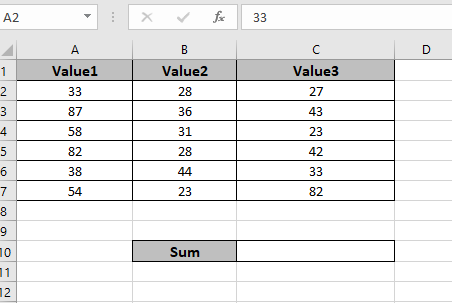In the above gif named range has been assigned and used in the formula. Follow the below steps to avoid having #NULL error.

1. First, select the range and name the range values via typing the values in the top left box.
2. Secondly, type the function name and open bracket symbol. =SUM(
3. Then type the exact name of the named range (  ) as it is case sensitive.
4. Excel will show you the suggestion on named range as you start typing the correct name for the range.
5. Press Enter to view the SUM.

As you can see the #NULL! Error can be handled when you use the correct Excel format while using range in the formula.

Hope this article about how Why #NULL! occur And How to Fix #NULL! error in Excel is explanatory. Find more articles on Excel error functions here. Please share your query below in the comment box. We will assist you.

Related Articles

#VALUE Error And How to Fix It in Excel

How to correct a #NUM! Error

How to use wildcards in excel

How to Remove Text in Excel Starting From a Position

Validation of text entries

Create drop down list in excel with colour

Remove leading and trailing spaces from text in Excel

Popular Articles

Edit a dropdown list

Absolute reference in Excel

If with conditional formatting

If with wildcards

Vlookup by date

Join first and last name in excel

Count cells which match either A or B

Convert Inches To Feet and Inches in Excel 2016

50 Excel Shortcut to Increase Your Productivity

Terms and Conditions of use

The applications/code on this site are distributed as is and without warranties or liability. In no event shall the owner of the copyrights, or the authors of the applications/code be liable for any loss of profit, any problems or any damage resulting from the use or evaluation of the applications/code.